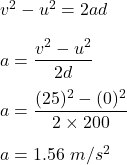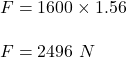## How large a net force is required to accelerate a 1600-kg SUV from rest to a speed of 25 m/s in a distance of 200 m

Question

How large a net force is required to accelerate a 1600-kg SUV from rest to a speed of 25 m/s in a distance of 200 m

in progress 0
2 months 2021-07-31T16:04:19+00:00 1 Answers 2 views 0

F=2496 N

Explanation:

Given that,

Mass of SUV, m = 1600 kg

Initial speed, u = 0

Final speed, v = 25 m/s

Distance, d = 200 m

We need to find the net force. Firstly, let’s find acceleration using equation of motion.Net force, F = maSo, the net force is 2496 N.